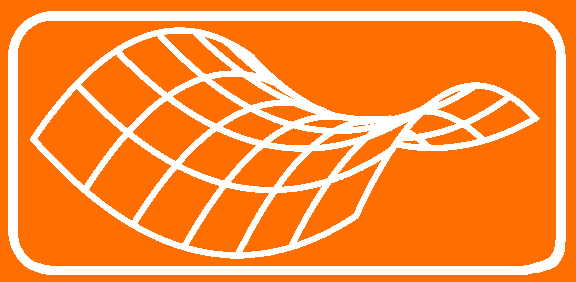Journal Home Page Cumulative Index List of all Volumes Complete Contentsof this Volume Previous Article Minimax Theory and its Applications 02 (2017), No. 2, 285--318Copyright Heldermann Verlag 2017 On a Minimax Problem for Ovals Rolf Walter Fakultät für Mathematik, Technische Universität, 44221 Dortmund, Germany rolf.walter@tu-dortmund.de [Abstract-pdf] For a bounded metric space $(X,d)$ we consider the quantity $$\delta(X) := \inf_{p\in X}\sup_{q \in X} d(p,q).$$ This purely metric invariant is known from approximation theory as the relative Chebyshev radius of $X$ w.r.t.\ $X$ itself. Despite its obvious meaning, the invariant $\delta(X)$ seems rather untouched in the geometric literature. Here we discuss, for a plane convex curve $X = \Gamma$, an isoperimetric type inequality between $\delta(\Gamma)$ and the perimeter $L(\Gamma)$, namely $L(\Gamma) \geq \pi \cdot \delta (\Gamma)$. Though the most general case is open there are classes of curves where definitive versions of the inequality are possible, including a discussion of equality. For quadrilaterals there is a surprising occurrence of `magic kites' as possible extremals. A finite algorithm for polygons is established, and numerous experiments with it yield strong support for a general validity of the inequality. Keywords: Metric invariant, relative Chebyshev radius, isoperimetric inequality. MSC: 51K05, 52A10, 52A40, 52-04, 53A04, 57Q55 [ Fulltext-pdf  (434  KB)] for subscribers only.